Get inspired by the success stories of our students in IIT JAM MS, ISI  MStat, CMI MSc DS.  Learn More

# ISI MStat PSB 2007 Problem 2 | Rank of a matrixThis is a very beautiful sample problem from ISI MStat PSB 2007 Problem 2 based on Rank of a matrix. Let's give it a try !!

## Problem- ISI MStat PSB 2007 Problem 2

Let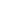and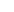be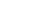real matrices such that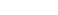and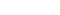Suppose that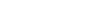is invertible. Show that rank(A)=rank(B).

### Prerequisites

Matrix Multiplication

Inverse of a matrix

Rank of a matrix

## Solution :

Here it is given thatis invertible which implies it's a non-singular matrix .

Now observe that ,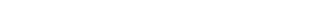asAgain ,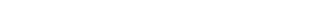as.

Now we know that for non-singular matrix M and another matrix N ,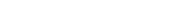. We will use it to get that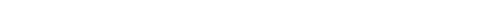and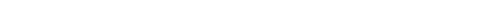.

And it's also known that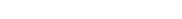. Hence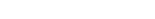(Proved) .

## Food For Thought

Try to prove the same using inequalities involving rank of a matrix.

## Subscribe to Cheenta at Youtube

This is a very beautiful sample problem from ISI MStat PSB 2007 Problem 2 based on Rank of a matrix. Let's give it a try !!

## Problem- ISI MStat PSB 2007 Problem 2

Letandbereal matrices such thatandSuppose thatis invertible. Show that rank(A)=rank(B).

### Prerequisites

Matrix Multiplication

Inverse of a matrix

Rank of a matrix

## Solution :

Here it is given thatis invertible which implies it's a non-singular matrix .

Now observe that ,asAgain ,as.

Now we know that for non-singular matrix M and another matrix N ,. We will use it to get thatand.

And it's also known that. Hence(Proved) .

## Food For Thought

Try to prove the same using inequalities involving rank of a matrix.

## Subscribe to Cheenta at Youtube

This site uses Akismet to reduce spam. Learn how your comment data is processed.

### Knowledge Partner